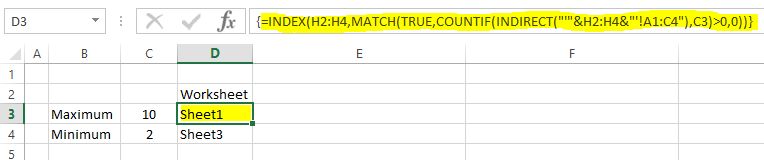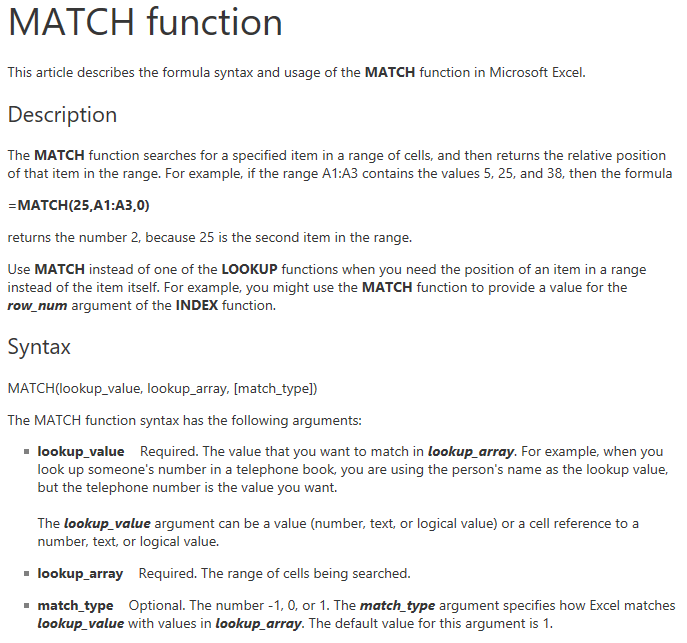# Formula Forensics No. 038 – Find Which Worksheet a Max or Min Value is located on

Recently, BrianB asked a question in the Chandoo.org Forums, “How to find a max/min value between sheets and return the sheet name as well?

I answered with an array formula
=INDEX(H2:H4,MATCH(TRUE,COUNTIF(INDIRECT(“‘”&H2:H4&”‘!A1:C4”),C3)>0,0))
which we will now pull apart and examine how it works.

As always at Formula Forensics we will use a sample file which you can use to follow along: Download Sample File

The sample file contains 3 worksheets of data Sheet1, Sheet2 and Sheet3 and a Results worksheet.

### Lets see how this works

Open the sample file

The sample data is located across 3 worksheets in cells A1:C4 with all cells containing the number 5
Sheet1!B2 has the maximum value of 10
Sheet3!B3 has the minimum value of 2We can find the Maximum value of the 3 areas using the Max() worksheet function and a multiple worksheet range using:

On the Results worksheet
C3: =MAX(Sheet1:Sheet3!A1:C4) Excel displays 10
C4: =MIN(Sheet1:Sheet3!A1:C4) Excel displays 2

Typically to find a cell which contains a value we would use an Index and compare the value to the Row/Column it is in.

But Index doesn’t have a 3rd Dimension to its lookup facilities.

So to find the worksheet that the Maximum or Minimum value is on we use a slight trick.

We use an array of the worksheets names as the input array to the Index
then check if the value is on that worksheets using a Countif() function.
This is all done as an array formula so that each worksheet is checked

This is shown as
=INDEX(H2:H4,MATCH(TRUE,COUNTIF(INDIRECT(“‘”&H2:H4&”‘!A1:C4”),C3)>0,0))
or
=INDEX(wsheets,MATCH(TRUE,COUNTIF(INDIRECT(“‘”&wsheets&”‘!A1:C4”),C3)>0,0))
where wsheets is a Named Formula for =Results!H2:H4

Note: Of course we can lookup any value, not just the Min or Max value.

Lets see how this works

Firstly list the worksheets in cells H2:H4In D3: enter the formula:
=INDEX(H2:H4,MATCH(TRUE,COUNTIF(INDIRECT(“‘”&H2:H4&”‘!A1:C4”),C3)>0,0)) press Ctrl+Shift+Enter
Excel will display Sheet1 as it is where the maximum value 10 is locatedIf we look at this formula we will see it is a simple Index() function (in Blue)
=INDEX(H2:H4,MATCH(TRUE,COUNTIF(INDIRECT(“‘”&H2:H4&”‘!A1:C4”),C3)>0,0))

where it has  H2:H4 as the Array and MATCH(TRUE,COUNTIF(INDIRECT(“‘”&H2:H4&”‘!A1:C4”),C3)>0,0) as the Row Number

H2:H4 is our list of worksheets
and
MATCH(TRUE,COUNTIF(INDIRECT(“‘”&H2:H4&”‘!A1:C4”),C3)>0,0) is returning the row number to lookup in the Array

### How does the Match function determine which row?

The function: MATCH(TRUE,COUNTIF(INDIRECT(“‘”&H2:H4&”‘!A1:C4”),C3)>0,0) uses the Excel Match() function.

The Match() function has the SyntaxSo in our example Match is looking for the lookup_value TRUE from the lookup_array COUNTIF(INDIRECT(“‘”&H2:H4&”‘!A1:C4”),C3)>0

What is COUNTIF(INDIRECT(“‘”&H2:H4&”‘!A1:C4”),C3)>0 doing

In English it says Count the number of times the Maximum value (C3) occurs in the Range A1:C4 on each worksheet and is it greater than 0?

If the Maximum value is on a worksheet the Countif() will return 1 or more if it is there multiple time and so the >0 will convert the Countif() value to True
If the Maximum value is not on a worksheet the Countif() will return 0 and so the >0 will convert the Countif() value to False

The Indirect() function takes the internal text and converts it to a properly constructed range

If you select the internal part of the Indirect() function
INDIRECT(“‘”&H2:H4&”‘!A1:C4”) then press F9
Excel will display {“‘Sheet1’!A1:C4″;”‘Sheet2’!A1:C4″;”‘Sheet3’!A1:C4”}
Showing that INDIRECT(“‘”&H2:H4&”‘!A1:C4”) is evaluating each of the 3 Ranges A1:C4 on each worksheet, Sheet1, Sheet2 & Sheet3

If you select the COUNTIF(INDIRECT(“‘”&H2:H4&”‘!A1:C4”),C3)>0 part of
=INDEX(H2:H4,MATCH(TRUE,COUNTIF(INDIRECT(“‘”&H2:H4&”‘!A1:C4”),C3)>0,0))
The press F9
Excel returns {TRUE;FALSE;FALSE}

The first true relates to the worksheet Sheet1 as it contains the maximum value it is true
The other 2 worksheets Sheet2 & Sheet3 don’t have the maximum value and so return False

So now:
=INDEX(H2:H4,MATCH(TRUE,COUNTIF(INDIRECT(“‘”&H2:H4&”‘!A1:C4”),C3)>0,0))

Simplifies to:
=INDEX(H2:H4,MATCH(TRUE,{TRUE;FALSE;FALSE},0))

The match will return 1 as the Value TRUE is found in the first Row of the {TRUE;FALSE;FALSE} array
and so
=INDEX(H2:H4,MATCH(TRUE,{TRUE;FALSE;FALSE},0))

Simplifies to:
=INDEX(H2:H4, 1)

which will return the first value in the H2:H4 range or Sheet1 as the result

You can examine the same formula in D4 where it uses named Formula wsheets instead of H2:H4 to find the worksheet that contains the minimum value.

## A Challenge

Can you solve the problem another way ?

Can you determine the cell address of the Max or Min across multiple worksheets?

Tomorrow, Formula Forensics 039 will discuss how to find the cell address of the Max or Min across multiple worksheets

## Other Posts in this Series

The Formula Forensics Series contains a wealth of useful solutions and information specifically about how Normal Formula and specifically Array Formula work.

http://chandoo.org/wp/formula-forensics-homepage/

If you have a formula and you want to understand how it works contact Hui and it may be featured in future posts.Hello Awesome...

My name is Chandoo. Thanks for dropping by. My mission is to make you awesome in Excel & your work. I live in Wellington, New Zealand. When I am not F9ing my formulas, I cycle, cook or play lego with my kids. Know more about me.

Thank you and see you around.

### Related articles:

 Written by Hui... Tags: advanced excel, countif(), INDEX(), INDIRECT(), Learn Excel, MATCH(), Microsoft Excel Formulas Home: Chandoo.org Main Page ? Doubt: Ask an Excel Question

### 4 Responses to “Formula Forensics No. 038 – Find Which Worksheet a Max or Min Value is located on”

1.Kevin Lehrbass says:

Very cool formula Hui! Thanks for sharing! Believe it or not I recently created a YouTube video about countif across sheets "Video 00139 Beyond 3D Formulas" https://youtu.be/3x-kQBIGRLA
Maybe this should be called "Advanced 3D Formula Week"

Cheers,
Kevin Lehrbass

2.XOR LX says:

Hi Hui.

You can also achieve the same without CSE and without volatility, viz:

=CEILING(MATCH(1,FREQUENCY(C3,Sheet1:Sheet3!\$A\$1:\$C\$4),0)/COUNT(Sheet1:Sheet3!\$A\$1:\$C\$4)
*COUNTA(wsheets),1)

Regards

3.XOR LX says:

Or, to give the full sheet name:

=INDEX(wsheets,CEILING(MATCH(1,FREQUENCY(C4,Sheet1:Sheet3!\$A\$1:\$C\$4),0) / COUNT(Sheet1:Sheet3!\$A\$1:\$C\$4)* COUNTA(wsheets),1))

Regards

4. […] writing this post I noticed that Hui over at Chandoo.org wrote a post about finding the max or min value across sheets. There are also some comments below the post with […]

 « CP046: Gantt charts & project planning using Excel Formula Forensics No. 039 – Find the Cell Address for a value (2D & 3D Reverse Lookup) »

### Get FREE Excel & Power-BI Newsletter

One email per week with Excel and Power BI goodness. Join 100,000+ others and get it free.